# NCERT Solutions for Class 9 Chapter 3

In this page we have NCERT Solutions for Class 9th Maths: Chapter 3 Coordinate Geometry Ex 3.1 ,3.2,3.3 for All exercise Hope you like them and do not forget to like , social share and comment at the end of the page.

## Chapter 3 Exercise 3.1 Solutions

Question 1
How will you describe the position of a table lamp on your study table to another person?
• We can use the concept of Coordinate Geometry to describe the position of a table lamp on the study table.  we have to take two lines, a perpendicular and horizontal.
• Considering the table as a plane and taking perpendicular line as Y axis and horizontal as X axis. Take one corner of table as origin where both X and Y axes intersect each other.
• Now, the length of table is Y axis and breadth is X axis. From The origin, join the line to the lamp and mark a point. Calculate the distance of this point from both X and Y axes and then write it in terms of coordinates.
• Let the distance of point from X axis is x and from Y axis is y then the position of the table lamp in terms of coordinates is (x, y).

Question 2
(Street Plan): A city has two main roads which cross each other at the centre of the city. These two roads are along the North-South direction and East-West direction.
All the other streets of the city run parallel to these roads and are 200 m apart. There are 5 streets in each direction. Using 1cm = 200 m, draw a model of the city on your notebook. Represent the roads/streets by single lines. There are many cross- streets in your model. A particular cross-street is made by two streets, one running in the North - South direction and another in the East - West direction. Each cross street is referred to in the following manner: If the 2nd street running in the North - South direction and 5th in the East - West direction meet at some crossing, then we will call this cross-street (2, 5). Using this convention, find:
(i) how many cross - streets can be referred to as (4, 3).
(ii) how many cross - streets can be referred to as (3, 4)
Let us taken X axis along S-N direction and Y axis along W-E direction. Now drawing the 5 lines representing roads parallel to X and Y axis each separated equally.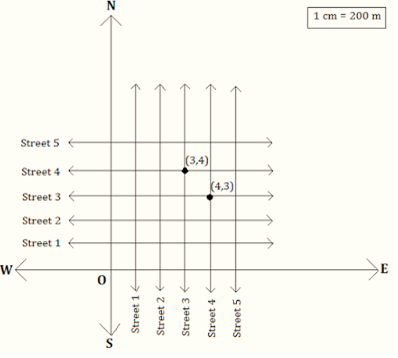(i) Only one street can be referred to as (4, 3) as we see from the figure.
(ii) Only one street can be referred to as (3, 4) as we see from the figure.

## Chapter 3 Exercise 3.2

Question 1
Write the answer of each of the following questions:
(i) What is the name of horizontal and the vertical lines drawn to determine the position of any point in the Cartesian plane?
(ii) What is the name of each part of the plane formed by these two lines?
(iii) Write the name of the point where these two lines intersect.
 What is the name of horizontal and the vertical lines drawn to determine the position of any point in the Cartesian plane? The name of horizontal lines and vertical lines drawn to determine the position of any point in the Cartesian plane is x-axis and y-axis respectively. What is the name of each part of the plane formed by these two lines? The name of each part of the plane formed by these two lines x-axis and y-axis is quadrants. Write the name of the point where these two lines intersect The point where these two lines intersect is called origin.

Question 2
Refer the below figure and write the following: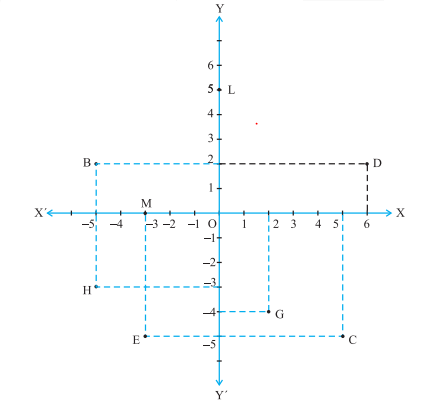(i) The coordinates of B.
(ii) The coordinates of C.
(iii) The point identified by the coordinates (-3, -5).
(iv) The point identified by the coordinates (2, -4).
(v) The abscissa of the point D.
(vi) The ordinate of the point H.
(vii)The coordinates of the point L.
(viii) The coordinates of the point M.
We can answer the above question by recalling these statements
(1) The distance of a point from y axis is called x –coordinate or abscissa and the distance of the point from x –axis is called y – coordinate or Ordinate
(2) The x-coordinate and y –coordinate of the point in the plane is written as (x, y) for point and is called the coordinates of the point
(3) A point on the x –axis has zero distance from x-axis so coordinate of any point on the x-axis will be (x, 0)
(4) A point on the y –axis has zero distance from y-axis so coordinate of any point on the y-axis will be (0, y)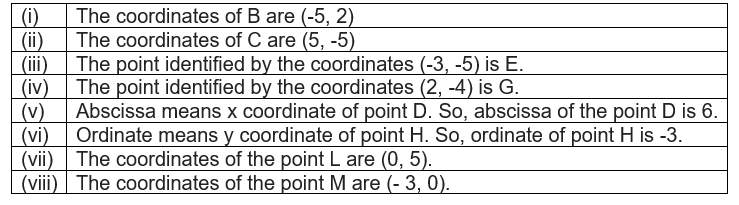## Chapter 3 Exercise 3.3

Question 1
In which quadrant or on which axis do each of the points (-2, 4), (3, -1), (-1, 0), (1, 2) and (-3, -5) lie? Verify your answer by locating them on the Cartesian plane.
We know the quadrant signs are given below

So on that basis we can derive the answer as

Now Let us draw the Cartesian plane as given below to verify the answer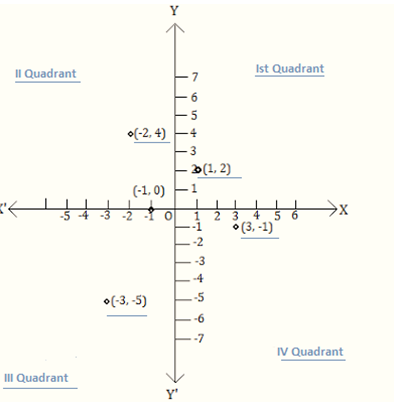Question 2
Plot the points (x, y) given in the following table on the plane, choosing suitable units of distance on the axes.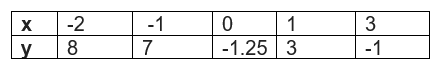We can draw the Cartesian plane by taking 1 unit as 1cm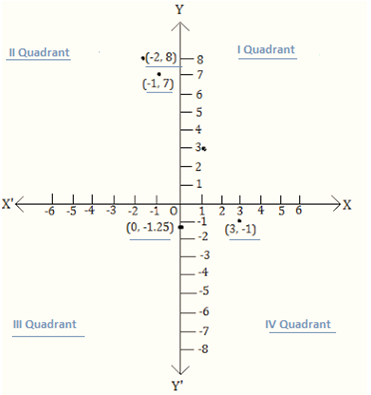## Summary

1. NCERT Solutions for Class 9th Maths: Chapter 3 has been prepared by Expert with utmost care. If you find any mistake.Please do provide feedback on mail. You can download the solutions as PDF in the below Link also
2. This chapter 3 has total 3 Exercise 3.1 ,3.2 and 3.3. This page conatins all the exercise in the chapter.